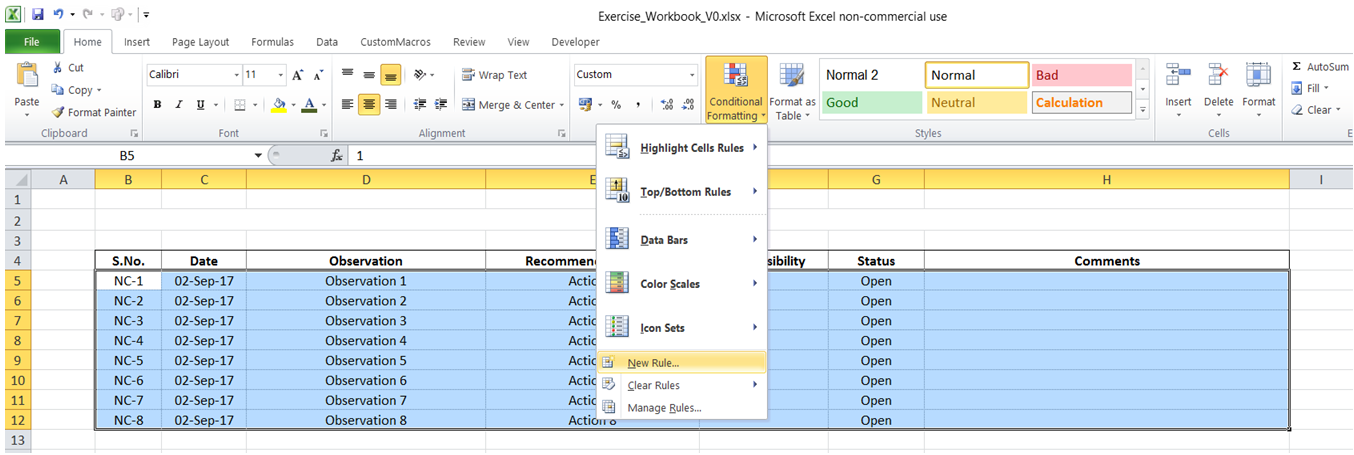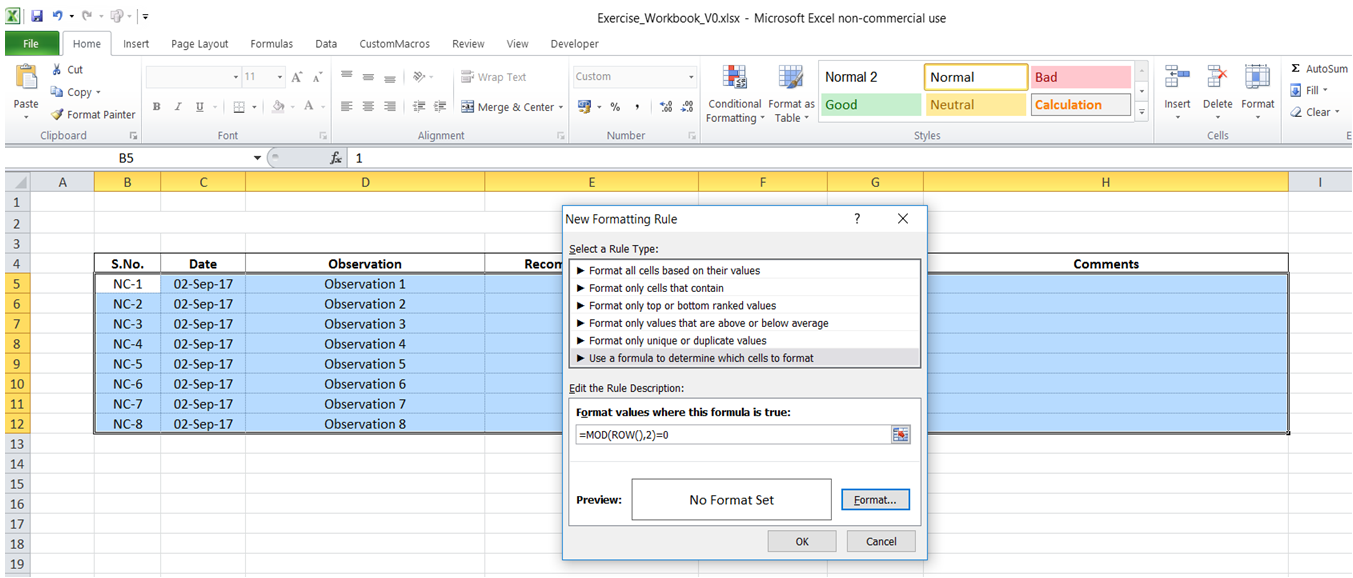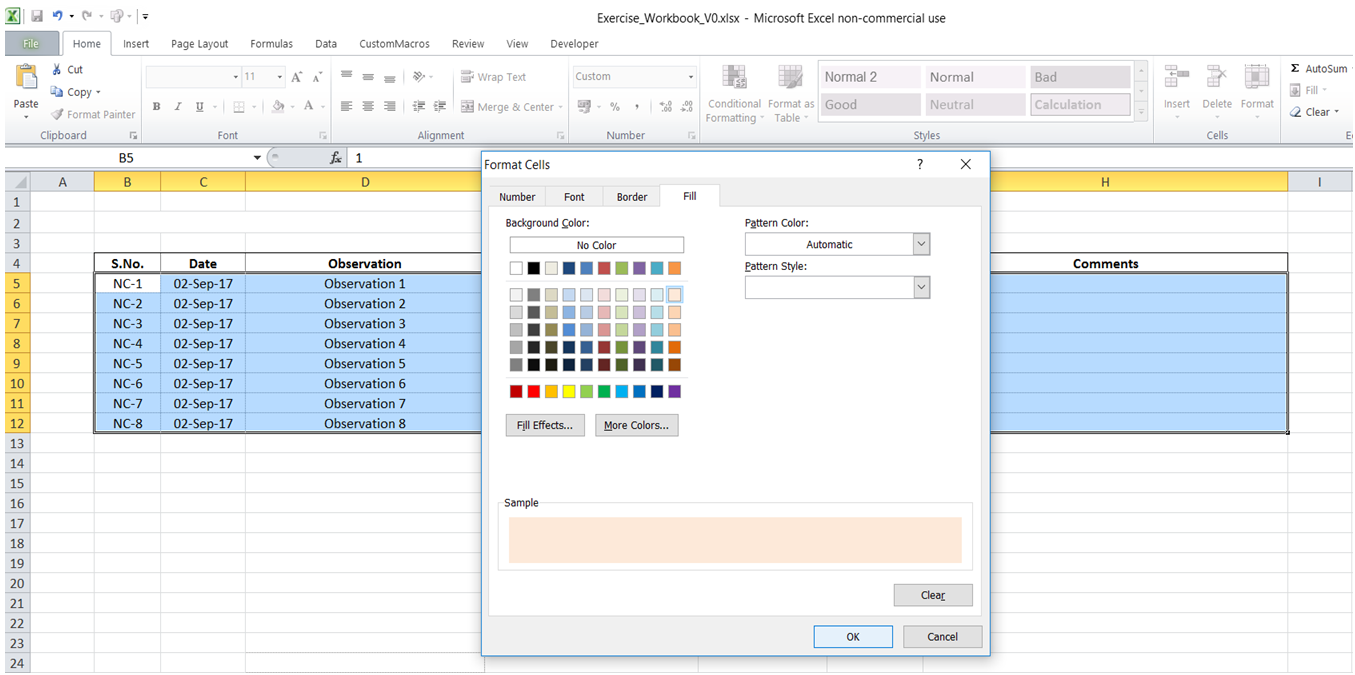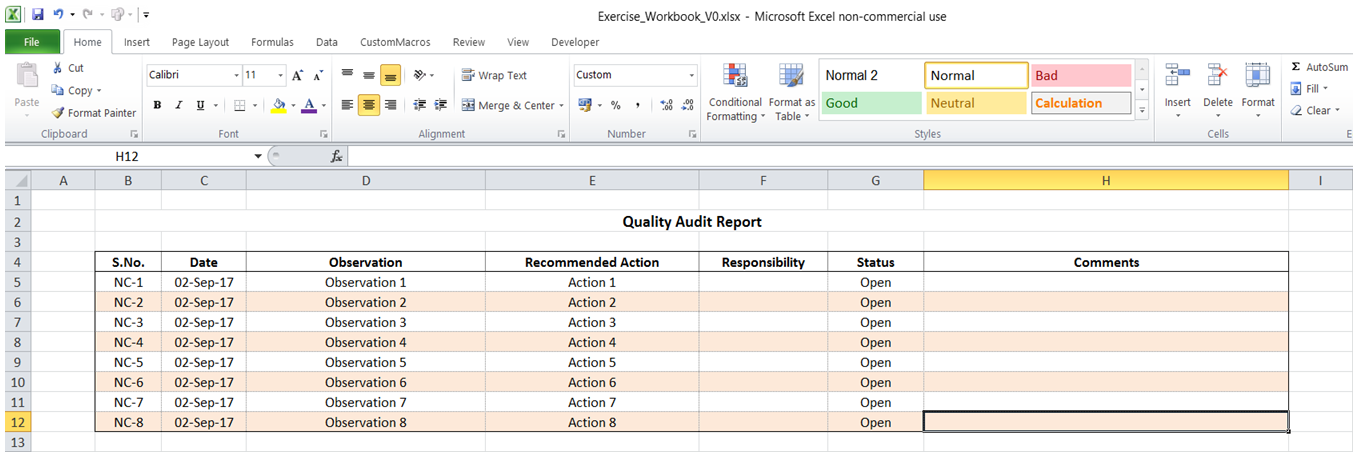## Alternate shade in data range in Excel

To do it in Excel, here is the answer:

a) Select the data range where you would like to apply alternate row shading. Under "Home" tab, click on "Conditional Formatting" -> "New Rule".b) Select "Use a formula to determine which cells to format". Enter =MOD(ROW(),2)=0 in the "Format values where this formula is true" field.

The formula selects all even numbered rows. In case odd numbered rows need to be selected, change the formula to =MOD(ROW(),2)=1.c) Click on Format-> Fill. Select color of your choice for shading. Click OK -> OK.d) The selected range would be formatted as required with alternate rows shaded.